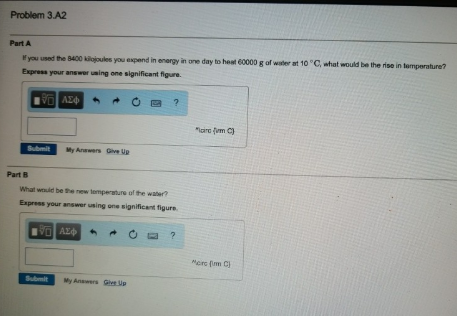# Problem: Part AIf you used the 8400 kilojoules you expend in energy in one day to heat 60000 g of water at 10°C, what would be the rise in temperature? Express your answer using one significant figure. Part BWhat would be the new temperature of the water? Express your answer using one significant figure.

###### FREE Expert Solution
96% (288 ratings)###### Problem Details

Part A

If you used the 8400 kilojoules you expend in energy in one day to heat 60000 g of water at 10°C, what would be the rise in temperature?

Part B

What would be the new temperature of the water?